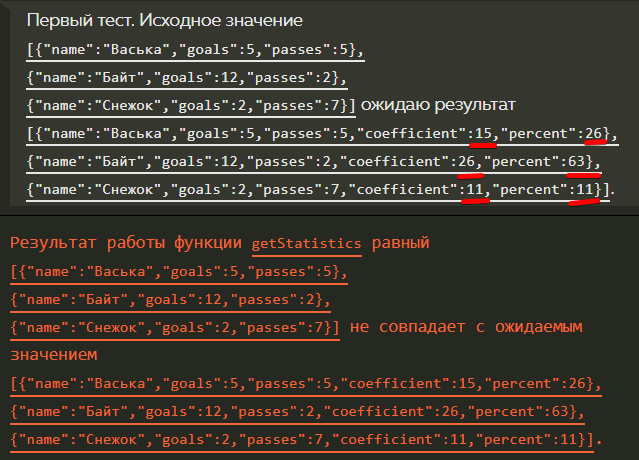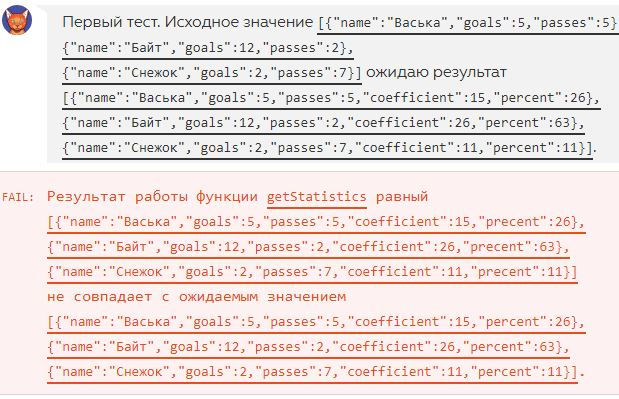# [20/28] Золотой мяч

Мой код
``````var getStatistics = function (players) {
var sumGoals = 0;
for (var i = 0; i < players.length; i++) {
sumGoals += players[i].goals;
for (var j = 0; j < players.length; j++) {
players[j].coefficient = players[j].goals * 2 + players[j].passes;
players[j].percent = Math.round(players[j].goals / sumGoals * 100);
}
}
return players;
};
``````
4 Симпатий

Это получается у вас вложенный цикл будет работать i раз, выполняя одно и то же, за исключением обновленного значения sumGoals и пересчета percent?

Я сделал пока так, но и это решение кажется не самым оптимальным.

Summary
``````var getStatistics = function (players) {
// Считаем сумму всех голов
var allGoals = 0;
for (var i = 0; i < players.length; i++) {
allGoals += players[i].goals;
}
// Добавляем новые свойства
for (var i = 0; i < players.length; i++) {
players[i].coefficient = players[i].goals * 2 + players[i].passes;
players[i].percent = Math.round((players[i].goals * 100) / allGoals);
}

return players;
};
``````
6 Симпатий

Может и не стоило мне вкладывать один цикл в другой. Ваш вариант мне кажется более логичным.

А у меня так вышло:

code
``````  var getStatistics = function (players) {
var totalGoals = 0;

players.forEach(function (player) {
totalGoals += player.goals;
});

players.forEach(function (player) {
player.coefficient = player.goals * 2 + player.passes;
player.percent = Math.round(player.goals * 100 / totalGoals);
});

return players;
};
``````

это получается все голы можно было так подсчитать, без перебора через цикл?Похоже на то. Отправьте код текстом, обязательно форматируйте его с помощью кнопки </> в редакторе сообщений и укажите версию системы и браузера, в котором проходите задание.

Браузер: Chrome Версия 64.0.3282.186 (Официальная сборка), (64 бит)
Система: macOS 10.13.3

``````var firstKicker = {
name: 'Миша',
goals: 0,
passes: 0,
coefficient: 0,
percent: 0
};
var secondKicker = {
name: 'Славон',
goals: 0,
passes: 0,
coefficient: 0,
percent: 0
};
var thirdKicker = {
name: 'Кирамзит',
goals: 0,
passes: 0,
coefficient: 0,
percent: 0
};

var kickers = [firstKicker,secondKicker,thirdKicker];

var getStatistics = function (players) {
return players;
};

getStatistics(kickers);``````

@juwain, ошибка проверкиСпасибо, передал разработчикам.

А это нормально, что система при проверке передает в исходных значениях полные данные, включая те, которые нужно рассчитать и добавить? Или ошибка?

На всякий случай - Win10 x64, Chrome 65.0.3325.181

Код
``````  var getStatistics = function (players) {
var totalGoals = 0;

for (var i = 0; i <= players.length - 1; i++) {
totalGoals += players[i].goals;
};

for (var i = 0; i <= players.length - 1; i++) {
players[i].coefficient = players[i].goals * 2 + players[i].passes;
players[i].percent = Math.round((players[i].goals * 100) / totalGoals);
}

return players;
};
``````

Нет, точно не нормально. Сейчас посмотрю, почему это происходит.

Ребят, кто-то может подсказать почему мой код не проходит проверку? Если запускаю сам, с теми же данными, то результаты такие же, как и при проверке системой.

Заранее спасибо за ответ!

`````` var firstPlayer = {
name: 'Васька',
goals: 5,
passes: 5
};

var secondPlayer = {
name: 'Байт',
goals: 12,
passes: 2
};

var thirdPlayer = {
name: 'Снежок',
goals: 2,
passes: 7
};

var soccerPlayers = [firstPlayer, secondPlayer, thirdPlayer];

var getStatistics = function (players) {
getCoefficient(soccerPlayers);
getPercent(soccerPlayers);
return players;
};

var getCoefficient = function(players) {
for (var i = 0; i < players.length; i++){
players[i].coefficient = (players[i].goals * 2) + players[i].passes;
}
}

var getPercent = function(players) {
var goalsSum = 0;
for (var i = 0; i < players.length; i++) {
goalsSum += players[i].goals;
}

for (var i = 0; i < players.length; i++) {
players[i].percent = Math.round((players[i].goals * 100) / goalsSum);
}
}

getStatistics(soccerPlayers);
``````попробуйте эту функцию в конец кода положить

Спасибо, но, к сожалению, не помоглоПохоже на какую-то ошибку в проверке.

getCoefficient(soccerPlayers);
getPercent(soccerPlayers);

функции нужно было запускать с параметром players. Моя ошибка, проморгал.

или я дурак , или лыжи не едут? Не понимаю почему мой код не проходит проверку??? ведь всё правильно работает) шо за ???
var getStatistics = function (players) {

Код
``````var getStatistics = function (players) {
var sumGoals = 0;
for (var j = 0; j < players.length; j++) {
sumGoals += players[j].goals;
}
for (var i = 0; i < players.length; i++) {
players[i].coefficient = players[i].goals * 2 + players[i].passes;
players[i].precent = Math.round(players[i].goals / sumGoals * 100);
}
return players;
};
``````
СкринВо балда я)))) написал имя свойства precent вместо percent…

Помогите плс
неправильно считаются проценты , что делать

var firstPlayer = {
name: ‘Васька’,
goals: 5,
passes: 5
};

var secondPlayer = {
name: ‘Байт’,
goals: 12,
passes: 2
};

var thirdPlayer = {
name: ‘Снежок’,
goals: 2,
passes: 7
};

var soccerPlayers = [firstPlayer, secondPlayer, thirdPlayer];

var getStatistics = function (players) {
var sumGoals = 0;
var thPercent = 0;
for (var i = 0; i < players[i].length; ++i){
sumGoals += players[i].goals;
}

for (var n = 0; n < players.length; ++n){
players[n].coefficient = (players[n].goals * 2) + players[n].passes;
thPercent = players[n].goals * 100 / sumGoals;
players[n].percent = Math.round(thPercent);
return players[n];
}

};
getStatistics(soccerPlayers);

Мой вариантЗолотой мяч
``````var getStatistics = function (players) {
var totalGoals = 0;
for (var i = 0; i < players.length; i++) {
players[i].coefficient = players[i].goals * 2 + players[i].passes;
totalGoals += players[i].goals;
}
for (i = 0; i < players.length; i++) {
players[i].percent = Math.round(players[i].goals * 100 / totalGoals);
}
return players;
};
``````

P.s. в принципе не особо отличается от решений выше…

2 Симпатий